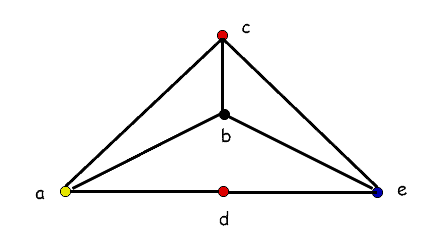## 1. Introduction

In this tutorial, we’ll talk about planar graphs and present some of their fundamental properties.

## 2. Planar Graphs

A planar graphis the one we can draw on the plane so that its edges don’t cross (except at nodes). A graph drawn in that way is also also known as a planar embedding or a plane graph.

So, there’s a difference between planar and plane graphs. A plane graph has no edge crossings, but a planar graph may be drawn without them. For example:is a planar but not a plane graph, whereasis a plane graph.is planar because it is isomorphic to.

### 2.1. Isomorphism

Formally, a planar graph is isomorphic to a plane graph.andare isomorphic graphs if there’s a 1-1 mappingof their vertices, and the following holds:For example:All three graphs are isomorphic to one another. The top left and the bottom graphs have the identity isomorphism (), whereas the isomorphism of the top right graph is.

## 3. Properties of Planar Graphs

Let’s check out some properties of planar graphs.

### 3.1. Faces

A graph defines connected regions on a plane we call faces, each of which is bordered by graph edges:Any face’s boundary contains the vertices and edges of the graphthat separate it from other regions.

One unbounded region, known as the exterior region, exists in every plane graph.

The number of edges constituting the boundary of a faceis its degree. In other terms, the length of the face’s boundary determines its degree.

For instance, the faces,, andofin the above image have the degree of.

### 3.2. Chromatic Number

As we recall from graph theory, the chromatic number of a graph is the least amount of colors necessary to color the vertices of a graph G such that no two adjacent nodes have the same color. Any planar graph’s chromatic number is always less than or equal to 4.

As a result, any planar graph always requires a maximum of four colors to color its vertices. For instance:This planar graph’s chromatic number is 4.

### 3.3. Proving a Graph Is (Not) Planar

There are several properties of planar graphs we can use in proofs:

1. If a connected planar graphhasedges andregions or faces then2. If a connected planar graphhasedges,vertices, andregions, then3. If a connected planar graphhasedges andvertices, then4. A complete graphis a planar iff5. A complete bipartite graphis planar iffor6. If and only if a subgraph of graphis homomorphic toor, thenis considered to be non-planar

A graph homomorphism is a mapping between two graphs that considers their structural differences. More precisely, a graphis homomorphic toif there’s a mappingsuch that. It maps vertices adjacent into vertices adjacent in.

The first three state the necessary conditions for planarity. For example, if we have a connected graph such that, we know it isn’t planar. However, necessary conditions aren’t sufficient, which means that some non-planar graphs can satisfy them. Therefore, we can use those properties to prove that a graph isn’t planar, not that it is.

The last property allows us to prove if a graph is planar. If we can’t findorin our graph, then we can be sure it’s planar.

### 3.4. Non-Planar Graphs

Non-planar graphs can’t be drawn in a plane without crossing edges.

Two well-known types of non-planar graphs are, a complete graph with 5 vertices, and, a complete bipartite graph with 3 vertices in each bipartition:The importance of these two graphs lies in the fact that any non-planar graph contains them as subgraphs.

## 4. Conclusion

In this article, we discussed planar graphs, one of the most widely used graph theory concepts.

A planar graph is a graph that can be drawn on a plane such that its edges intersect only at their end nodes. A plane graph is a graph whose edges don’t intersect. So, we call a graph planar if we can draw it as a plane graph.

To prove that a graph is planar, we need to show that it doesn’t contain the complete graphor the complete bipartite graph.